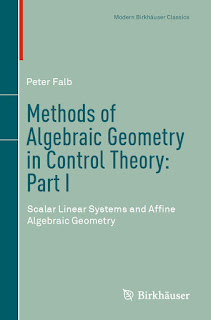ALGEBRA MATHEMATICS

# Methods of Algebraic Geometry in Control Theory Part I Scalar Linear Systems and Affine Algebraic Geometry PDF Free DownloadAn introduction to the ideas of algebraic geometry in the motivated context of system theory.” Thus the author describes his textbook that has been specifically written to serve the needs of students of systems and control. Without sacrificing mathematical care, the author makes the basic ideas of algebraic geometry accessible to engineers and applied scientists. The emphasis is on constructive methods and clarity rather than abstraction. The student will find here a clear presentation with an applied flavor, of the core ideas in the algebra-geometric treatment of scalar linear system theory. The author introduces the four representations of a scalar linear system and establishes the major results of a similar theory for multivariable systems appearing in a succeeding volume (Part II: Multivariable Linear Systems and Projective Algebraic Geometry).